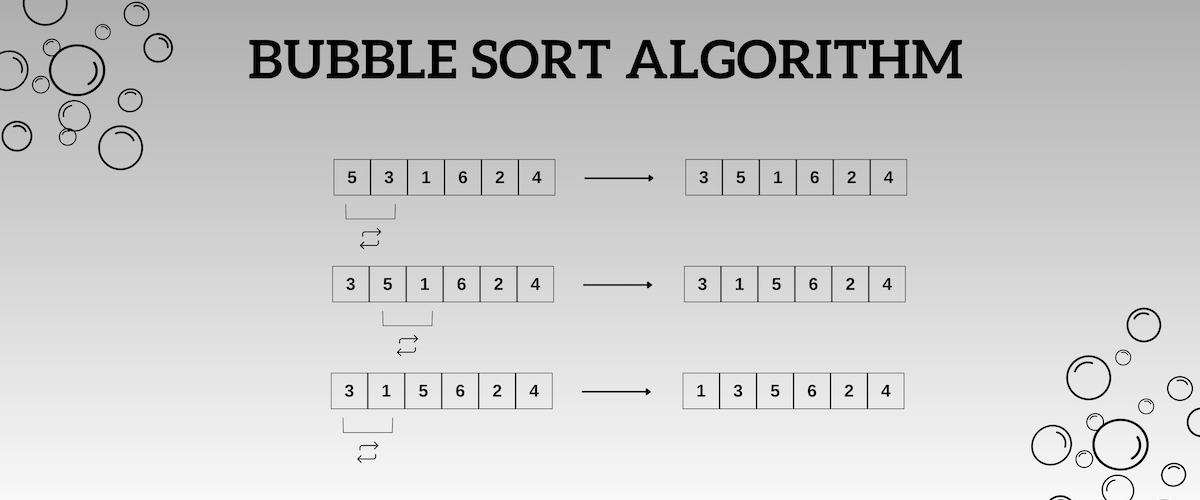19/06/2023

# Bubble Sort AlgorithmBubble sort is a simple sorting algorithm that works by repeatedly comparing adjacent elements and swapping them if they are in the wrong order. The algorithm starts at the beginning of the array and compares the first two elements. If the first element is greater than the second element, they are swapped.

The algorithm then moves on to the next two elements and repeats the process. This continues until the end of the array is reached.

## Does Bubble Sort Algorithm Efficient

The bubble sort algorithm is a very simple algorithm to understand and implement. However, it is not very efficient for large arrays. The worst-case time complexity of bubble sort is O(n2), where n is the number of elements in the array. This means that the time it takes to sort the array increases quadratically as the number of elements increases.

Bubble sort is not a very good algorithm to use for sorting large arrays. However, it can be useful for sorting small arrays or for educational purposes.

## Step-by-step Bubble Sort Algorithm Explanation

Here’s a step-by-step explanation of the Bubble Sort algorithm:

2. Compare the first element with the second element. If the first element is greater than the second element, swap them.
3. Move to the next pair of elements (2nd and 3rd), and continue comparing and swapping until the end of the list is reached.
4. At this point, the largest element is in its correct position at the end of the list.
5. Repeat steps 2-4 for all other elements, except the last one.
6. After each iteration, the next largest element will be in its correct position at the end of the list.
7. Continue the iterations until the entire list is sorted.

Let’s say we have an unsorted list: [5, 3, 8, 2, 1]

### 1st Iteration

• Comparing 5 and 3: Swap [3, 5, 8, 2, 1]
• Comparing 5 and 8: No swap [3, 5, 8, 2, 1]
• Comparing 8 and 2: Swap [3, 5, 2, 8, 1]
• Comparing 8 and 1: Swap [3, 5, 2, 1, 8]

Result: [3, 5, 2, 1, 8]

### 2nd iteration

• Comparing 3 and 5: No swap [3, 5, 2, 1, 8]
• Comparing 5 and 2: Swap [3, 2, 5, 1, 8]
• Comparing 5 and 1: Swap [3, 2, 1, 5, 8]

Result: [3, 2, 1, 5, 8]

### 3rd iteration

• Comparing 3 and 2: Swap [2, 3, 1, 5, 8]
• Comparing 3 and 1: Swap [2, 1, 3, 5, 8]

Result: [2, 1, 3, 5, 8]

### 4th iteration

• Comparing 2 and 1: Swap [1, 2, 3, 5, 8]

Result: [1, 2, 3, 5, 8]

## Bubble Sort Algorithm Usage Areas

Bubble sort is a simple sorting algorithm that repeatedly steps through a list of elements and compares adjacent elements, swapping them if they are in the wrong order. While bubble sort is not efficient for large data sets, it can still find its usage in certain scenarios where simplicity and ease of implementation are prioritized over efficiency. Here are some areas where bubble sort may be used:

1. Educational Purposes: Bubble sort is often used in computer science and programming courses as an introductory sorting algorithm. Its straightforward implementation helps beginners understand the concept of sorting and algorithm analysis.
2. Small Data Sets: Bubble sort can be suitable for sorting small data sets, such as arrays or lists with only a few elements. In such cases, the performance difference between bubble sort and more complex algorithms is negligible.
3. Partially Sorted Data: If the input data is already partially sorted, bubble sort can be relatively efficient. It has a best-case time complexity of O(n) when the input is already sorted, as it only requires a single pass to confirm that the list is sorted.
4. Online Sorting: Bubble sort can be used in situations where new elements are continuously added to an already sorted list. Since bubble sort performs comparisons and swaps adjacent elements, it can easily accommodate new elements while maintaining the sort order.
5. Teaching and Demonstrating Sorting Algorithms: Bubble sort is often used to illustrate the concept of sorting algorithms and their performance characteristics. It provides a simple and visual way to demonstrate how sorting algorithms work and how their efficiency can vary.

## Bubble Sort Algorithm in Programming Languages

The bubble sort algorithm can be implemented in any programming language.

### Python Bubble Sort Algorithm

``````def bubble_sort(arr):
n = len(arr)

# Traverse through all array elements
for i in range(n):
# Last i elements are already in place
for j in range(0, n - i - 1):

# Traverse the array from 0 to n-i-1
# Swap if the element found is greater than the next element
if arr[j] > arr[j + 1]:
arr[j], arr[j + 1] = arr[j + 1], arr[j]
return arr

# Example usage:
array = [5, 3, 8, 2, 1]
print(bubble_sort(array))
``````

### Javascript Bubble Sort Algorithm

``````function bubbleSort(arr) {
var len = arr.length;
var swapped;

do {
swapped = false;

for (var i = 0; i < len - 1; i++) {
if (arr[i] > arr[i + 1]) {
// Swap elements
var temp = arr[i];
arr[i] = arr[i + 1];
arr[i + 1] = temp;
swapped = true;
}
}
// Optimized: Reduce the number of iterations on each pass
len--;
} while (swapped);

return arr;
}
// Example usage:
var array = [5, 3, 8, 2, 1];
console.log(bubbleSort(array));
``````